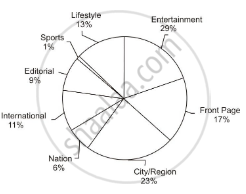# If the Total Number of Words Printed in the 'International' Section Was Approximately 88770 - Mathematics

#### Question

MCQ

Direction :  Answer the question on the basis of the information given below.

The pie chart given below shows the percentage of print space allocation of articles printed on different sections of a newspaper.If the total number of words printed in the 'International' section was approximately 88770, then which of the following is the closest approximation of the total number of words printed in the 'Lifestyle' section?

• 57000

• 105000

• 210000

• 175000

#### Solution

105000

Explanation:

because if 88700 is the number of words printed in the 'International' section, which is same as 11 % of the total print material, then the total number of words in the 'Lifestyle' section can be calculated as follows:

88770/11 xx 13 = 104810 ≈ 105000

Therefore, 105000 is the closest approximation of the required answer.

Concept: Graph and Charts (Entrance Exam)
Is there an error in this question or solution?